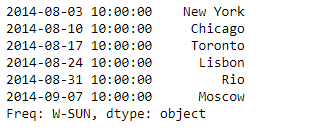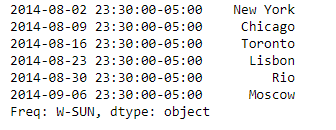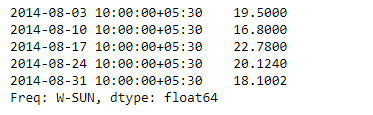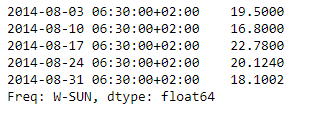# Python | Pandas Series.tz_convert

Python is a great language for doing data analysis, primarily because of the fantastic ecosystem of data-centric python packages. Pandas is one of those packages and makes importing and analyzing data much easier.

Pandas series is a One-dimensional ndarray with axis labels. The labels need not be unique but must be a hashable type. The object supports both integer- and label-based indexing and provides a host of methods for performing operations involving the index.

Pandas` Series.tz_convert()` function works with time zone aware indexes. It convert tz-aware axis to target time zone.

Syntax: Series.tz_convert(tz, axis=0, level=None, copy=True)

Parameter :
tz : string or pytz.timezone object
axis : the axis to convert
level : int, str, default None
copy : Also make a copy of the underlying data.

Returns : Series

Example #1: Use `Series.tz_convert()` function to convert the time zone aware index of the given Series to the target time zone.

 `# importing pandas as pd ` `import` `pandas as pd ` ` `  `# Creating the Series ` `sr ``=` `pd.Series([``'New York'``, ``'Chicago'``, ``'Toronto'``, ``'Lisbon'``, ``'Rio'``, ``'Moscow'``]) ` ` `  `# Create the Datetime Index ` `didx ``=` `pd.DatetimeIndex(start ``=``'2014-08-01 10:00'``, freq ``=``'W'``,  ` `                           ``periods ``=` `6``, tz ``=` `'Asia/Calcutta'``)  ` ` `  `# set the index ` `sr.index ``=` `didx ` ` `  `# Print the series ` `print``(sr) `

Output :Now we will use `Series.tz_convert()` function to convert the given time zone index to time zone aware index to the target time zone which is ‘US/Central’.

 `# convert to 'US / Central' ` `sr.tz_convert(``'US/Central'``) `

Output :As we can see in the output, the `Series.tz_convert()` function has converted the time zone of the index of the given series object to the desired time zone.

Example #2: Use `Series.tz_convert()` function to convert the time zone aware index of the given Series to the target time zone.

 `# importing pandas as pd ` `import` `pandas as pd ` ` `  `# Creating the Series ` `sr ``=` `pd.Series([``19.5``, ``16.8``, ``22.78``, ``20.124``, ``18.1002``]) ` ` `  `# Create the Datetime Index ` `didx ``=` `pd.DatetimeIndex(start ``=``'2014-08-01 10:00'``, freq ``=``'W'``,  ` `                     ``periods ``=` `5``, tz ``=` `'Asia/Calcutta'``)  ` ` `  `# set the index ` `sr.index ``=` `didx ` ` `  `# Print the series ` `print``(sr) `

Output :Now we will use `Series.tz_convert()` function to convert the given time zone index to time zone aware index to the target time zone which is ‘Europe/Berlin’

 `# convert to 'Europe / Berlin' ` `sr.tz_convert(``'Europe/Berlin'``) `

Output :My Personal Notes arrow_drop_upCheck out this Author's contributed articles.

If you like GeeksforGeeks and would like to contribute, you can also write an article using contribute.geeksforgeeks.org or mail your article to contribute@geeksforgeeks.org. See your article appearing on the GeeksforGeeks main page and help other Geeks.

Please Improve this article if you find anything incorrect by clicking on the "Improve Article" button below.

Article Tags :

1

Please write to us at contribute@geeksforgeeks.org to report any issue with the above content.